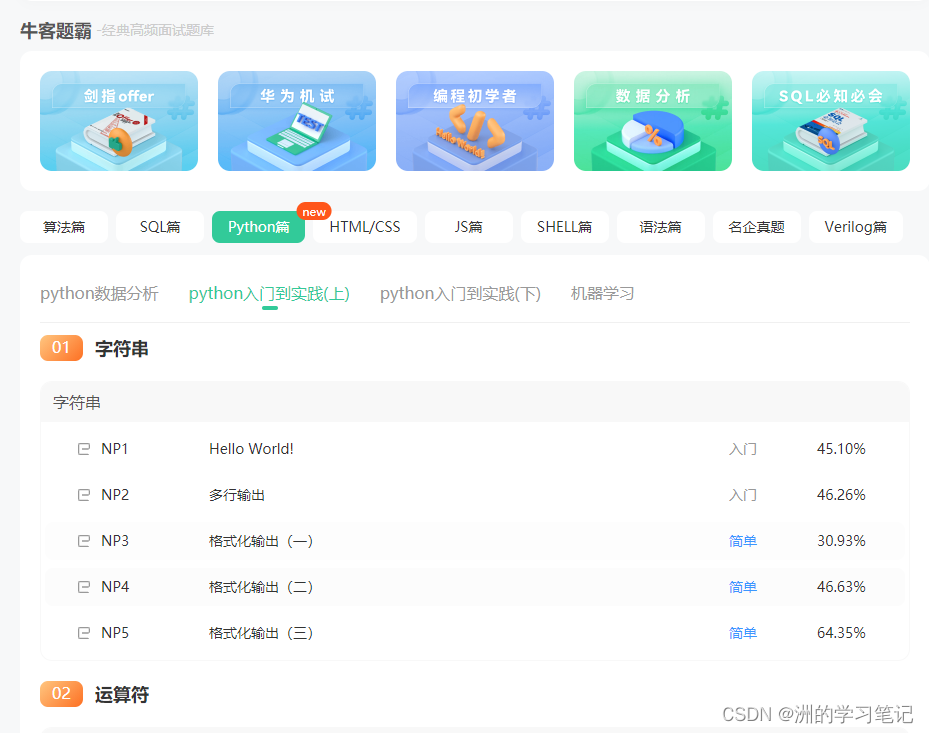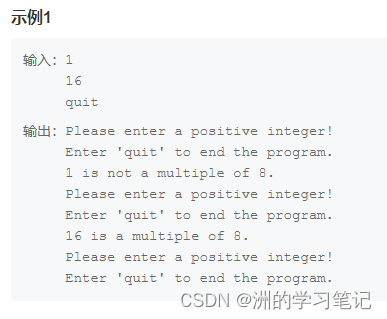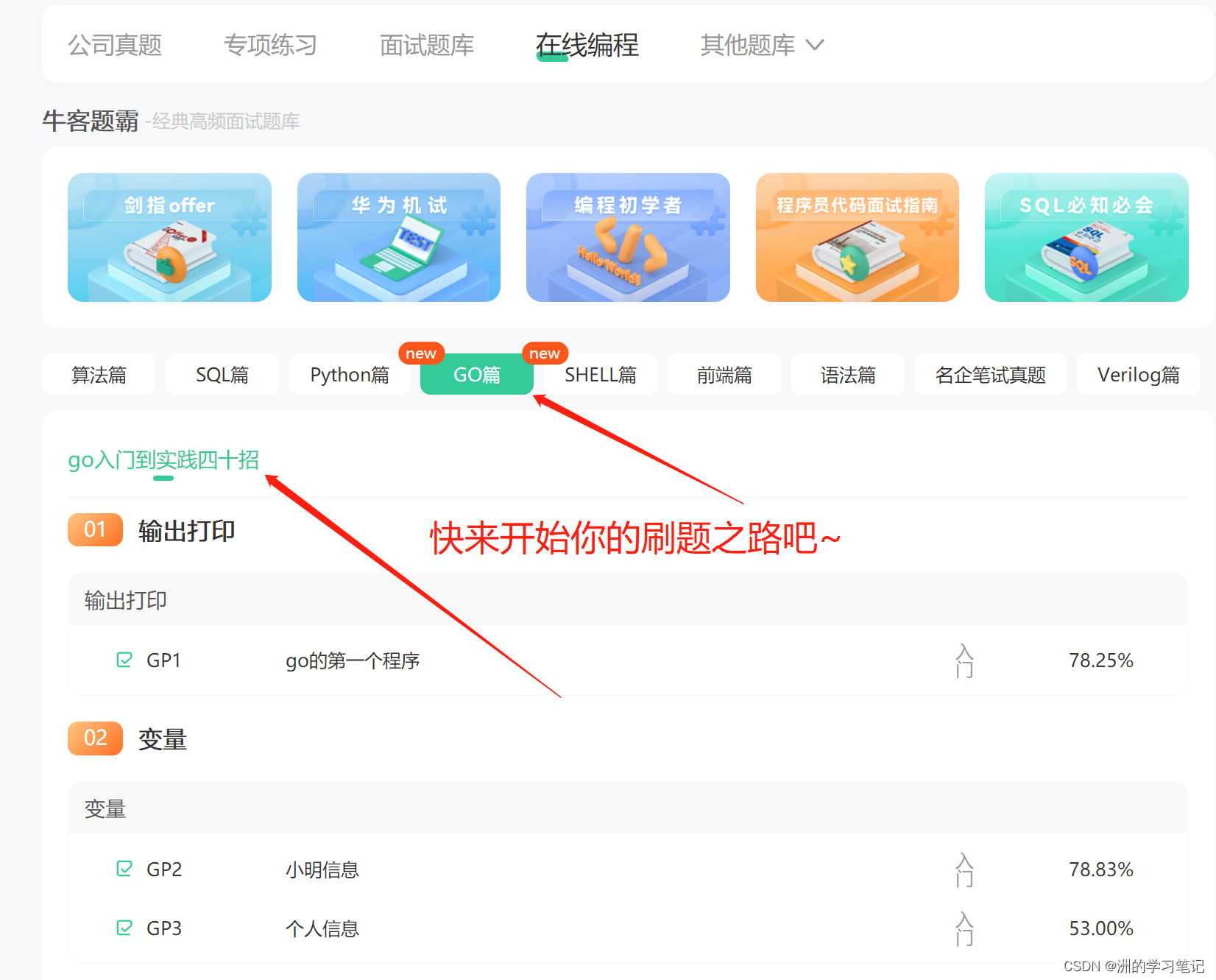出租广告位,需要合作请联系站长

+关注

# 前言

- 本期是Python从0到入门4|字典复习、函数入门、综合实践，有不懂的地方可以评论进行讨论！

• 接下来我将继续更新Python刷题系列、数据库从0到入门刷题系列等等。
• 本系列文章采用牛客的核心代码模式进行案例代码提供，帮助大家从0到入门的学习过程中进行配套的刷题~
• 推荐给大家一款刷题、面试的神器，我也是用这一款神器进行学习的！~链接如下：刷题神器跳转链接
• 该神器不仅网页界面十分美观，而且操作起来极易上手入门！非常适合新手进行系统性的学习！
• 小白新手可以通过该神器进行日常的刷题、看大厂面经、学习计算机基础知识、与大牛面对面沟通~ 刷题的图片已经放在下面了~# Q1：字典复习-首都

``````按题目描述进行输出即可。
Beijing is the capital of China!
Moscow is the capital of Russia!
Paris is the capital of France!
``````

``````cities_dict = {'Beijing': {'Capital': 'China'},'Moscow': {'Capital': 'Russia'},'Paris': {'Capital': 'France'}}
for i in sorted(cities_dict.keys()):
print('%s is the capital of %s!'%(i,cities_dict[i]['Capital']))
``````

# Q2：函数入门-求差

``````方法一：
x = int(input())
y = int(input())

def cal(x1, y1):
return x1 - y1

print(cal(x, y))
print(cal(y, x))

lambda 传入参数： 传回结果 f = lambda x, y: x - y 等价于
def f(x, y):
return x - y

cal = lambda x, y : x-y
x = int(input())
y = int(input())
print(cal(x, y))
print(cal(y, x))
``````

# Q3：函数入门-牛牛的朋友

[‘Niu Ke Le’, ‘Niumei’, ‘Niuneng’, ‘GOLO’]
[0, 1, 2, 3]

``````friends_list = ['Niu Ke Le', 'Niumei', 'Niuneng', 'GOLO']
print(friends_list)

def replace(list, index):
list[index] = index

for a in range(len(friends_list)):
replace(friends_list, a)

print(friends_list)
``````

# Q4：综合案例：自动售卖饮料机

What kind of drink would you like?

``````print("What kind of drink would you like?")
kind_of_drink = input()
if kind_of_drink in "cola":
else:
print("The", kind_of_drink, "has been sold out!")
``````

# Q5：综合案例-被8整除的数字``````active = True
while(active):
print("Please enter a positive integer!\nEnter 'quit' to end the program.")
try:
resp = input()
if resp == 'quit':
active = False
else:
resp = int(resp)
if resp<1 or resp >100:
print('数字合法')
continue
if resp%8 == 0:
print(f'{resp} is a multiple of 8.')
else:
print(f'{resp} is not a multiple of 8.')
except TypeError:
continue

``````

# Q6：综合案例-披萨店的订单

``````按题目描述进行输出即可。
['durian', 'bacon', 'chichen']
``````

``````pizza_orders = ['chichen', 'bacon', 'durian']
finished_pizza = []

while len(pizza_orders) > 0:
pizza = pizza_orders[-1]
pizza_orders.pop(-1)
finished_pizza.append(pizza)

print(finished_pizza)
``````

# 总结

1、算法篇（398题）：面试必刷100题、算法入门、面试高频榜单
2、数据结构篇（300题）：都是非常经典的链表、树、堆、栈、队列、动态规划等
3、语言篇（500题）：C/C++、java、python入门算法练习
4、SQL篇（82题）：快速入门、SQL必知必会、SQL进阶挑战、面试真题
5、大厂笔试真题：字节跳动、美团、百度、腾讯…掌握经验不在惧怕面试！2 0

pdf(new) 更多>